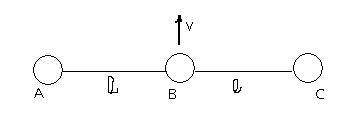# collisions

Three identical balls are connected by light inextensible strings with each other as shown and rest over a smooth horizontal table. At the moment t=0, ball B is imparted a velocity. Calculate the velocity of A when it collides with C.•Abhishek Priyam ·

lol..

i cahanged answer thrice.. did calculation msitake..

Now by conservation of momentum when A and C collides... velcoity of B=v0/3

By conservation of energy

mv02/2=1/2m(v0/3)2+2mx2/2

x is speed of A and C

x= 2v0/3

where Vo/3 is velocity in upward direction... so right componetn=v0/√3

•satan92 ·

if not clear how velocity v0/3

it is by conservation of momentum

finally when distance between A and C=0

then for the length to be L the velocities of all of them must be same

hence

mv0=3mv'

•Abhishek Priyam ·

tanx for giving the explanation... mere ko aaram ho gaya..  

•Shreyan ·

oh...got it..thanx..
even i did silly wala mistake!!! 

•RAJIVKUMAR SINGH ·

What would be the trajectory followed by A

•